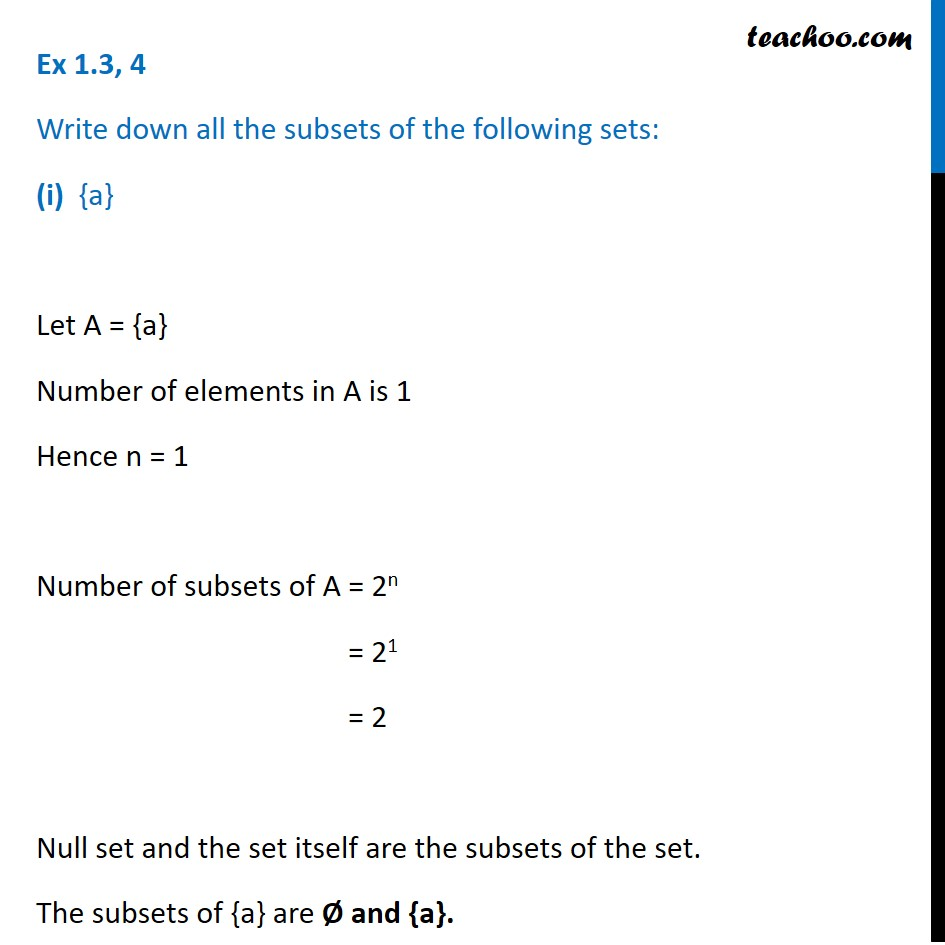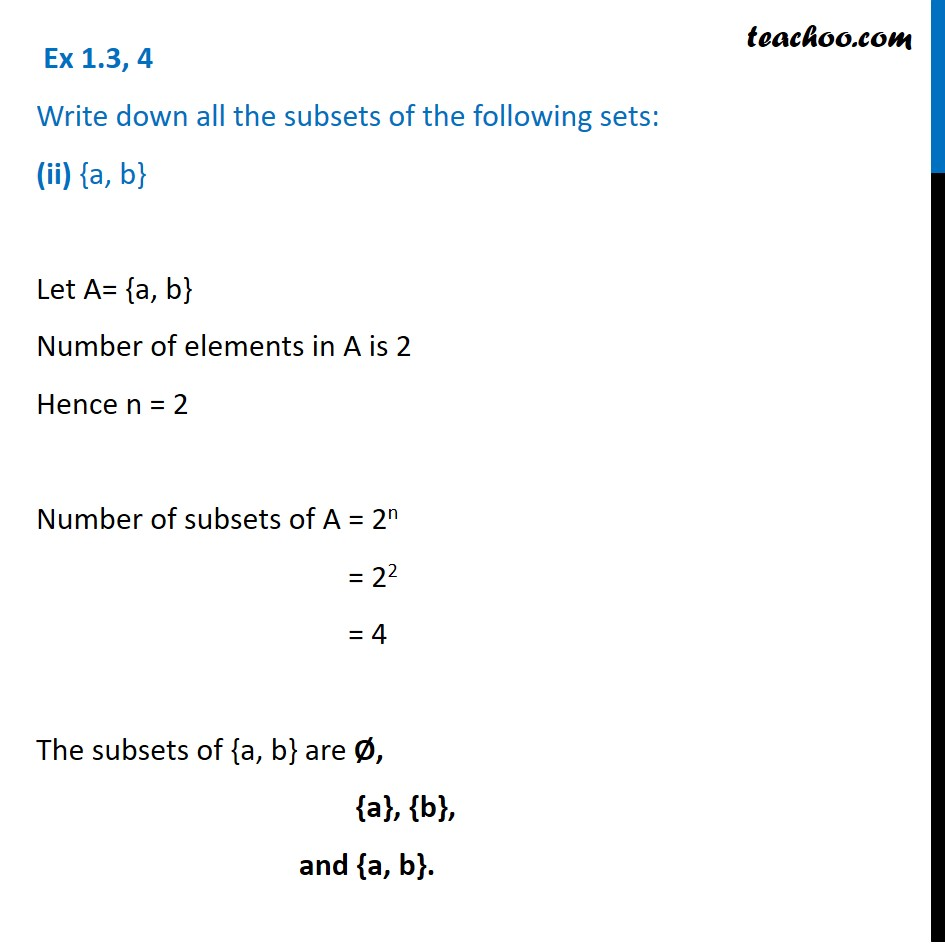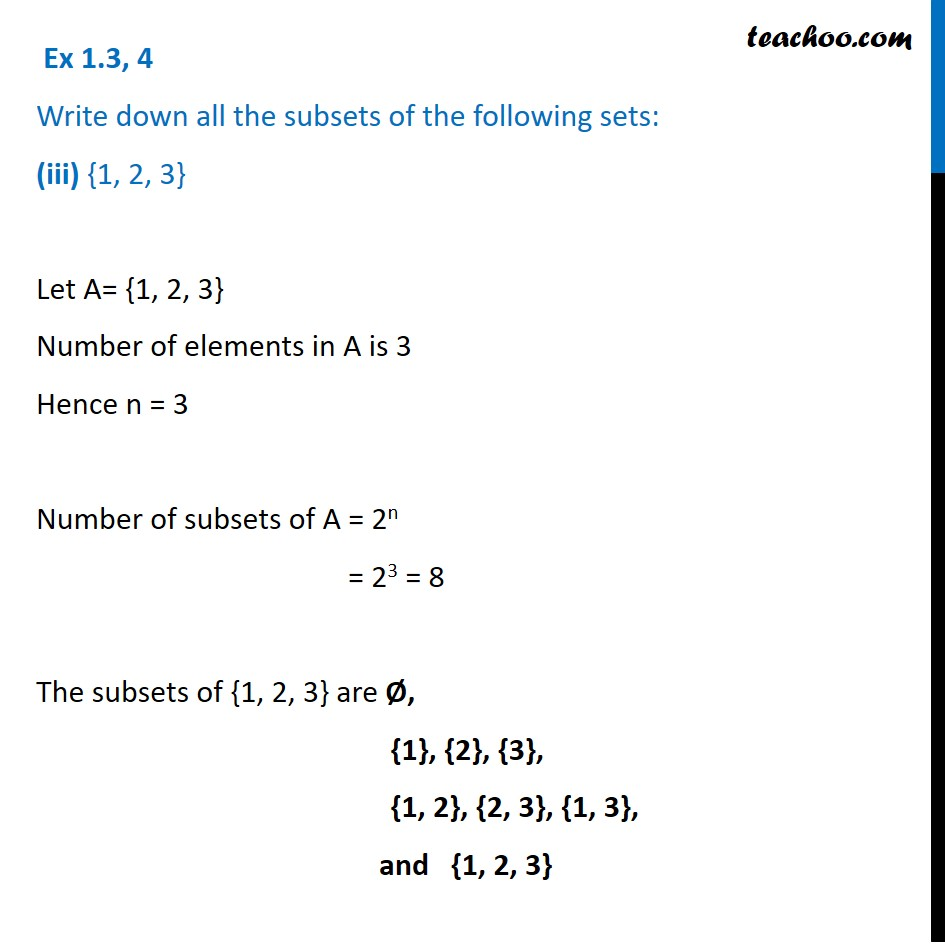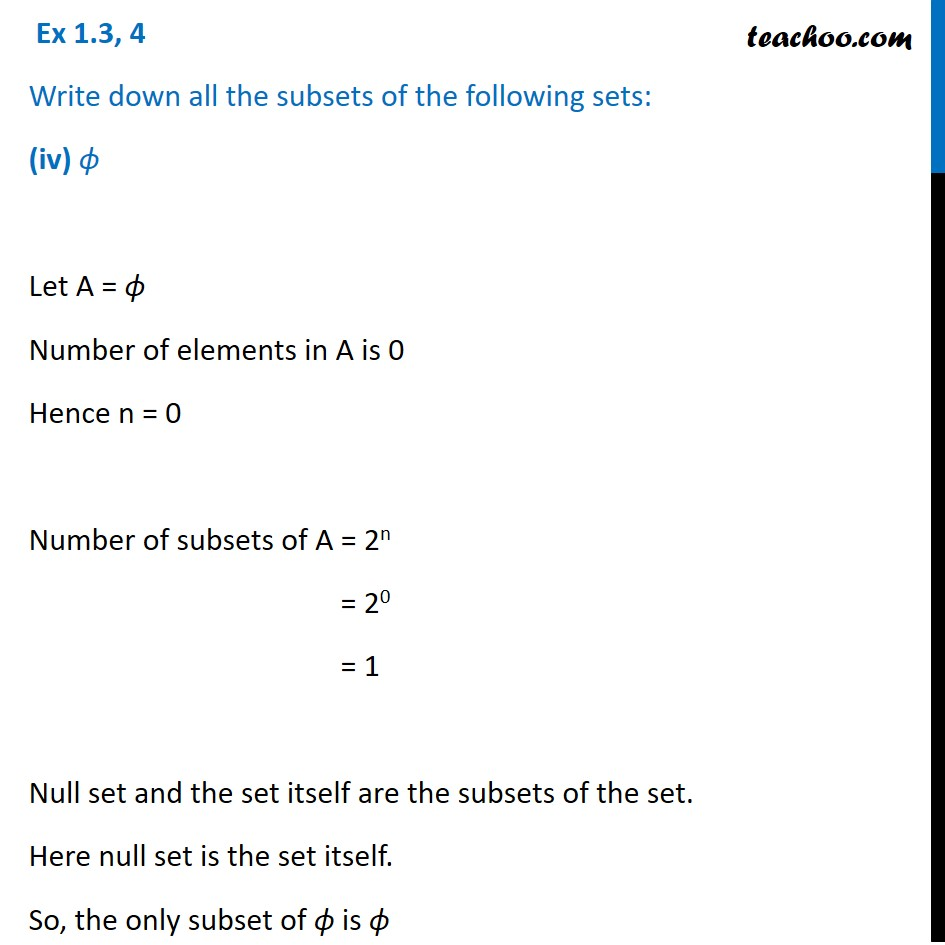1. Chapter 1 Class 11 Sets
2. Concept wise
3. Power Set

Transcript

Ex 1.3, 4 Write down all the subsets of the following sets: (i) {a} Let A = {a} Number of elements in A is 1 Hence n = 1 Number of subsets of A = 2n = 21 = 2 Null set and the set itself are the subsets of the set. The subsets of {a} are Ø and {a}. Ex 1.3, 4 Write down all the subsets of the following sets: (ii) {a, b} Let A= {a, b} Number of elements in A is 2 Hence n = 2 Number of subsets of A = 2n = 22 = 4 The subsets of {a, b} are Ø, {a}, {b}, and {a, b}. Ex 1.3, 4 Write down all the subsets of the following sets: (iii) {1, 2, 3} Let A= {1, 2, 3} Number of elements in A is 3 Hence n = 3 Number of subsets of A = 2n = 23 = 8 The subsets of {1, 2, 3} are Ø, {1}, {2}, {3}, {1, 2}, {2, 3}, {1, 3}, and {1, 2, 3} Ex 1.3, 4 Write down all the subsets of the following sets: (iv) ϕ Let A = ϕ Number of elements in A is 0 Hence n = 0 Number of subsets of A = 2n = 20 = 1 Null set and the set itself are the subsets of the set. Here null set is the set itself. So, the only subset of ϕ is ϕ

Power Set